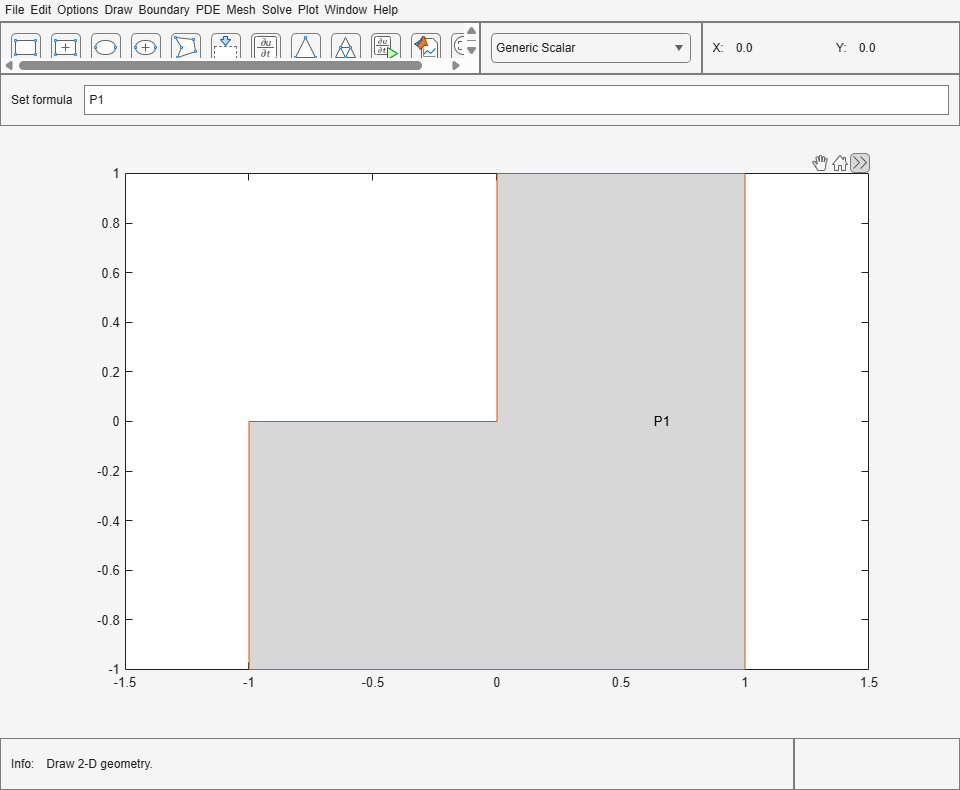# pdepoly

Draw polygon in PDE Modeler app

## Syntax

``pdepoly(X,Y)``
``pdepoly(X,Y,label)``

## Description

example

````pdepoly(X,Y)` draws a polygon with the corner coordinates (vertices) defined by `X` and `Y`. The `pdepoly` command opens the PDE Modeler app with the specified polygon drawn in it. If the app is already open, `pdepoly` adds the specified polygon to the app window without deleting any existing shapes.`pdepoly` updates the state of the geometry description matrix inside the PDE Modeler app to include the polygon. You can export the geometry description matrix from the PDE Modeler app to the MATLAB® Workspace by selecting DrawExport Geometry Description, Set Formula, Labels.... For details on the format of the geometry description matrix, see `decsg`.```

example

````pdepoly(X,Y,label)` assigns a name to the polygon. Otherwise, `pdepoly` uses a default name, such as `P1`, `P2`, and so on.```

## Examples

collapse all

Open the PDE Modeler app window containing a polygon representing the L-shaped membrane geometry.

`pdepoly([-1 0 0 1 1 -1],[0 0 1 1 -1 -1])`

Call the `pdepoly` command again to draw the diamond-shaped region with corners in `(0.5,0)`, `(1,-0.5)`, `(0.5,-1)`, and `(0,-0.5)`. The `pdepoly` command adds the second polygon to the app window without deleting the first.

`pdepoly([0.5 1 0.5 0],[0 -0.5 -1 -0.5])`Open the PDE Modeler app window with a polygon representing the L-shaped membrane geometry. Assign the name `L-shaped-membrane` to this polygon.

`pdepoly([-1 0 0 1 1 -1],[0 0 1 1 -1 -1],'L-shaped-membrane')`## Input Arguments

collapse all

x-coordinates of vertices defining the polygon, specified as a vector of real numbers.

Example: ```pdepoly([-1 0 0 1 1 -1],[0 0 1 1 -1 -1])```

Data Types: `double`

y-coordinates of vertices defining the polygon, specified as a vector of real numbers.

Example: ```pdepoly([-1 0 0 1 1 -1],[0 0 1 1 -1 -1])```

Data Types: `double`

Name of the polygon, specified as a character vector or string scalar.

Data Types: `char` | `string`

## Tips

• `pdepoly` opens the PDE Modeler app and draws a polygon. If, instead, you want to draw polygons in a MATLAB figure, use the `plot` function, for example:

```x = [-1,-0.5,-0.5,0,1.5,-0.5,-1]; y = [-1,-1,-0.5,0,0.5,0.9,-1]; plot(x,y,'.-')```